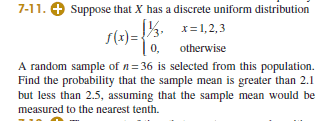# Uniform Discrete Sample Distribution

## Homework Statement2. Homework Equations [/B]

So the sample mean is 2. the sample variance would be [[(3-1+1)-1]/12]/36 = 8/432.

## The Attempt at a Solution

Is it, P[ (2.1-2)/sqrt(8/432) < z < (2.5-2)/sqrt(8/432)] = 0.232574.

The book answer is 0.2312. I just want to be sure.

Last edited:

## Answers and Replies

vela
Staff Emeritus
Science Advisor
Homework Helper
Education Advisor
Your calculation looks fine, but I get a different numerical answer than you do. I get 0.2311.

It must be due to my rounding, I am using the Z table from the back of my book so I lose precision I guess.

vela
Staff Emeritus
Science Advisor
Homework Helper
Education Advisor
It shouldn't be off by that much, though. What values of ##z## did you get and the corresponding values from the table?

vela
Staff Emeritus
Science Advisor
Homework Helper
Education Advisor
I see what probably happened. You're rounding the values of ##z## to two decimal places, right? When you use the tables, you need to interpolate to get a more accurate answer.

Ray Vickson
Science Advisor
Homework Helper
Dearly Missed

## Homework Statement2. Homework Equations [/B]

So the sample mean is 2. the sample variance would be [[(3-1+1)-1]/12]/36 = 8/432.

## The Attempt at a Solution

Is it, P[ (2.1-2)/sqrt(8/432) < z < (2.5-2)/sqrt(8/432)] = 0.232574.

The book answer is 0.2312. I just want to be sure.

Using the computer algebra package Maple (instead of tables) I get the answer = 0.2310970815 ≈ 0.2311. However, this is an approximation, based on use of the normal distribution to get the distribution of the sum. We can also get an exact answer (by looking at the probability generating function for the sum). Since ##2.1 < \sum_{i=1}^{36} X_i \,/36 < 2.5## we have ## 75.6 < \sum X_i < 90##, so (interpreting the inequalities as strict, the way they are written), the sum ##\sum X_i## must lie between 76 and 89. Therefore, with ##S \equiv \sum_{i=1}^{36} X_i## we have:
$$\text{exact answer} = \sum_{k=76}^{89} P(S = k) = 0.2379271979 \approx 0.2379$$

Oh, well then. I will know to interpolate next time around from the tables. Thanks for the help.